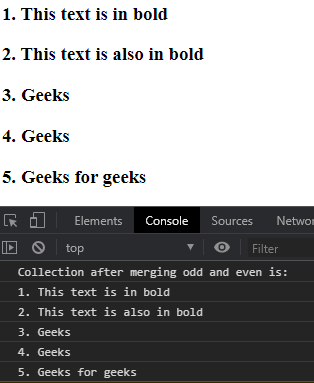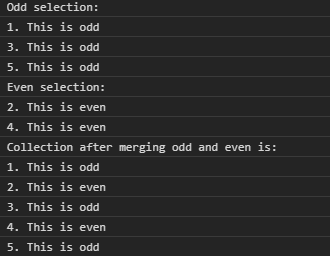# D3.js selection.merge() Function

• Last Updated : 23 Aug, 2020

The selection.merge() function in d3.js is used to merge the two given selections into one and return the new selection after merging them. The selection which is returned has the same number of groups and the same parents as this selection.

Syntax:

`selection.merge(other);`

Parameters: This function accepts single parameter other that describe selection is to be merged.

Return Values: This function returns a selection.

Example 1:

## HTML

 ```<``html` `lang``=``"en"``>`` ` `<``head``>``    ``<``meta` `charset``=``"UTF-8"``>``    ``<``meta` `name``=``"viewport"` `path1tent="``width``=``device``-width, ``                    ``initial-scale``=``1``.0">``    ``<``script` `src``=``"https://d3js.org/d3.v4.min.js"``>``    ````    ``<``script` `src``=``        ``"https://d3js.org/d3-selection.v1.min.js"``>``    `````` ` `<``body``>``    ``<``div``>``        ``<``h3``>1. This text is in bold``        ``<``h3``>2. This text is also in bold``        ``<``h3``>3. Geeks``        ``<``h3``>4. Geeks``        ``<``h3``>5. Geeks for geeks``    ```` ` `    ``<``script``>``        ``// Filtering odd children``        ``const odd = d3.selectAll("h3")``            ``.select(function (d, i) {``                ``return i & 1 ? this : null;``            ``});`` ` `        ``// Filtering even children``        ``const even = d3.selectAll("h3")``            ``.select(function (d, i) {``                ``return i & 1 ? null : this;``            ``});``             ` `        ``// Merging both selections``        ``const merged = odd.merge(even).nodes();`` ` `        ``// Printing text content``        ``console.log(``"Collection after merging odd and even is:")``        ``merged.forEach((e) => {``            ``console.log(e.textContent);``        ``});``    `````` ` ``

Output:Example 2:

## HTML

 ```<``html` `lang``=``"en"``>`` ` `<``head``>``    ``<``meta` `charset``=``"UTF-8"``>``    ``<``meta` `name``=``"viewport"` `path1tent="``width``=``device``-width, ``                    ``initial-scale``=``1``.0">`` ` `    ``<``script` `src``=``"https://d3js.org/d3.v4.min.js"``>``    ````    ``<``script` `src``=``        ``"https://d3js.org/d3-selection.v1.min.js"``>``    `````` ` `<``body``>``    ``<``div``>``        ``<``h5``>1. This is odd``        ``<``h5``>2. This is even``        ``<``h5``>3. This is odd``        ``<``h5``>4. This is even``        ``<``h5``>5. This is odd``    ```` ` `    ``<``script``>``        ``// Filtering odd children``        ``var even = d3.selectAll("h5")``            ``.select(function (d, i) {``                ``return i & 1 ? this : null``            ``});`` ` `        ``// Filtering even children``        ``var odd = d3.selectAll("h5")``            ``.select(function (d, i) {``                ``return i & 1 ? null : this``            ``});`` ` `        ``// Merging both selections``        ``const merged = odd.merge(even).nodes();``        ``even = even.nodes();``        ``odd = odd.nodes();``        ``console.log("Odd selection: ")``        ``odd.forEach((e) => {``            ``console.log(e.textContent);``        ``});``        ``console.log("Even selection: ")``        ``even.forEach((e) => {``            ``console.log(e.textContent);``        ``});``        ``// Printing text content``        ``console.log(``"Collection after merging odd and even is:")``        ``merged.forEach((e) => {``            ``console.log(e.textContent);``        ``});``    `````` ` ``

Output:My Personal Notes arrow_drop_up